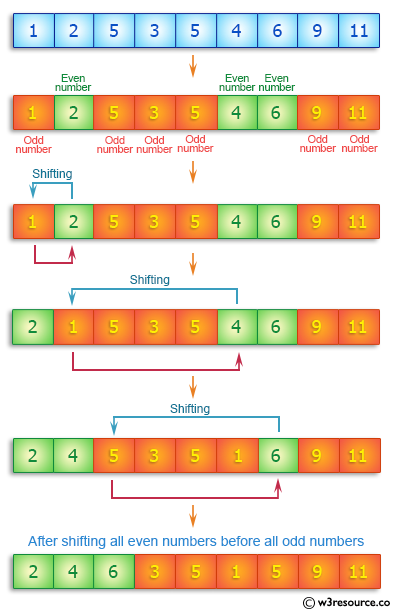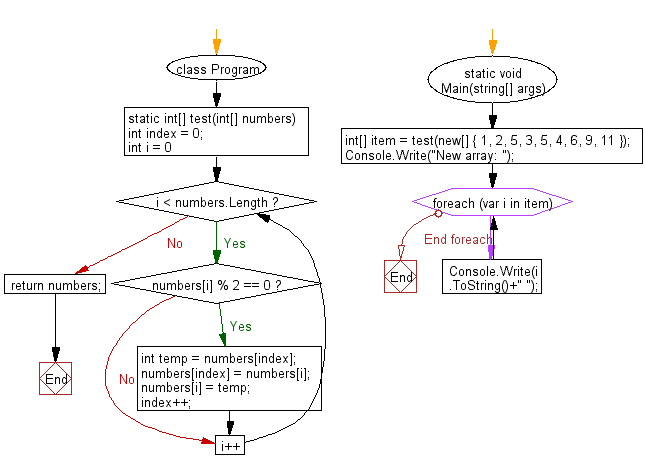﻿ C# - Create a new array by shifting even numbers before odd# C# Sharp Basic Algorithm Exercises: Create new array from a given array of integers shifting all even numbers before all odd numbers

## C# Sharp Basic Algorithm: Exercise-132 with Solution

Write a C# Sharp program to create new array from a given array of integers shifting all even numbers before all odd numbers.

Pictorial Presentation:Sample Solution:

C# Sharp Code:

``````using System;
namespace exercises
{
class Program
{
static void Main(string[] args)
{
int[] item = test(new[] { 1, 2, 5, 3, 5, 4, 6, 9, 11 });
Console.Write("New array: ");
foreach(var i in item)
{
Console.Write(i.ToString()+" ");
}
}
static int[] test(int[] numbers)
{
int index = 0;
for (int i = 0; i < numbers.Length; i++)
{
if (numbers[i] % 2 == 0)
{
int temp = numbers[index];
numbers[index] = numbers[i];
numbers[i] = temp;

index++;
}
}
return numbers;
}
}
}
```
```

Sample Output:

`New array: 2 4 6 3 5 1 5 9 11`

Flowchart:C# Sharp Code Editor:

Improve this sample solution and post your code through Disqus

What is the difficulty level of this exercise?

Test your Programming skills with w3resource's quiz.

﻿